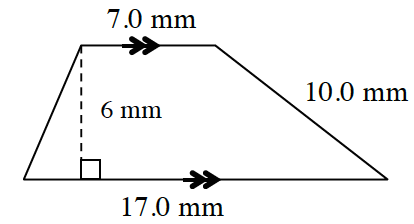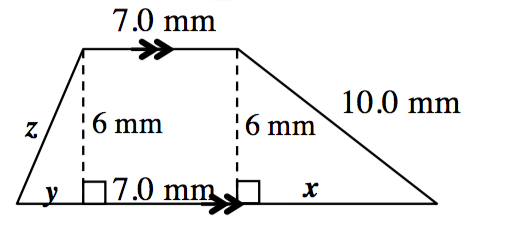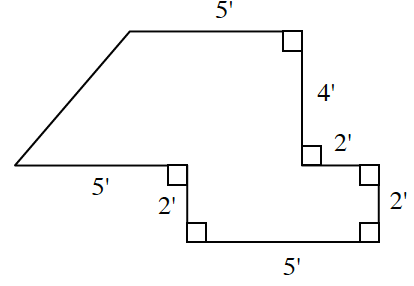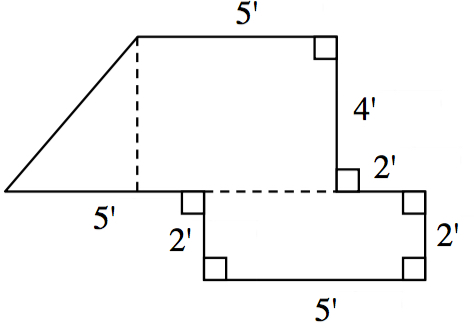### Home > INT2 > Chapter 6 > Lesson 6.2.2 > Problem6-63

6-63.

Calculate the area and perimeter of each polygon below. Show all work.

1.Look at the diagram below.
First solve for $x$, then $y$, then $z$.$\text{P }= 2\sqrt{10} + 34 \approx 40.3\text{ mm, A }= 72 \text{ sq. mm}$

1.Start by determining the length of each dashed segment in the diagram below.